Next: Our attack Up: Cycling attack against faulty Previous: Cycling attack against faulty

## Fast exponentiation algorithms

Suppose that you have to compute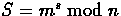. If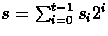is the binary decomposition of s , then this computation can efficiently be performed as depicted on Fig. 3. Indeed, if we respectively letand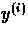the values of z and y at step i (Lines 5 and 7 of Fig. 3), we have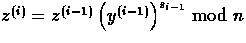and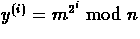. So,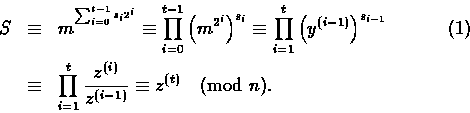Figure 3: Square-and-multiply method (I).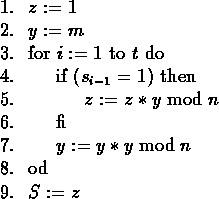Figure 4: Square-and-multiply method (II).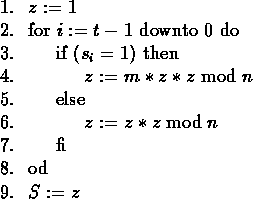Scanning the bits of exponent s from left to right, we obtain another efficient algorithm to compute(see Fig. 4). The drawback in this algorithm is that it cannot easily be parallelized. Consequently, we only focus on the first algorithm.